# MPI topic: Point-to-point

##### Experimental html version of Parallel Programming in MPI, OpenMP, and PETSc by Victor Eijkhout. download the textbook at https:/theartofhpc.com/pcse
$\newcommand\inv{^{-1}}\newcommand\invt{^{-t}} \newcommand\bbP{\mathbb{P}} \newcommand\bbR{\mathbb{R}} \newcommand\defined{ \mathrel{\lower 5pt \hbox{{\equiv\atop\mathrm{D}}}}}$ 4.1 : Blocking point-to-point operations
4.1.1 : Example: ping-pong
4.1.2 : Send call
4.1.4 : Problems with blocking communication
4.1.4.2 : Eager vs rendezvous protocol
4.1.4.3 : Serialization
4.1.6 : Pairwise exchange
4.2 : Nonblocking point-to-point operations
4.2.1 : Nonblocking send and receive calls
4.2.2 : Request completion: wait calls
4.2.2.1 : Wait for one request
4.2.2.2 : Wait for all requests
4.2.2.3 : Wait for any requests
4.2.2.4 : Polling with MPI Wait any
4.2.2.5 : Wait for some requests
4.2.2.6 : Receive status of the wait calls
4.2.2.7 : Latency hiding / overlapping communication and computation
4.2.2.8 : Buffer issues in nonblocking communication
4.2.3 : Wait and test calls
4.3 : The Status object and wildcards
4.3.1 : Source
4.3.2 : Tag
4.3.3 : Error
4.3.4 : Count
4.3.5 : Example: receiving from any source
4.4 : More about point-to-point communication
4.4.1 : Message probing
4.4.2 : Errors
4.4.3 : Message envelope
4.5 : Review questions

# 4 MPI topic: Point-to-point

## 4.1 Blocking point-to-point operations

crumb trail: > mpi-ptp > Blocking point-to-point operations

Suppose you have an array of numbers $x_i\colon i=0,\ldots,N$ and you want to compute \begin{equation} y_i=(x_{i-1}+x_i+x_{i+1})/3\colon i=1,…,N-1. \end{equation} As seen in figure  2.6  , we give each processor a contiguous subset of the $x_i$s and $y_i$s. Let's define $i_p$ as the first index of $y$ that is computed by processor $p$. (What is the last index computed by processor $p$? How many indices are computed on that processor?)

We often talk about the owner computes model of parallel computing: each processor owns' certain data items, and it computes their value. The values used for this computation need of course not be local, and this is where the need for communication arises.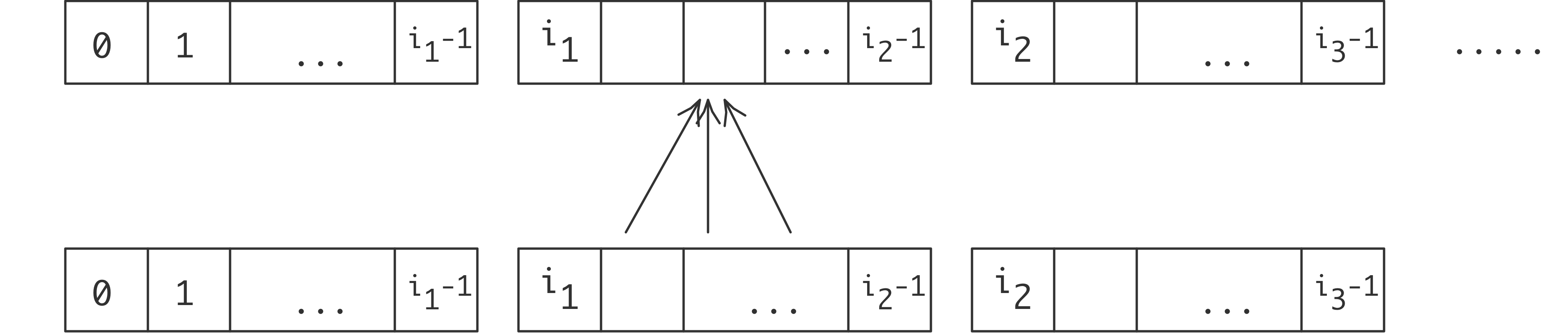FIGURE 4.1: Three point averaging in parallel, case of interior points

Let's investigate how processor $p$ goes about computing $y_i$ for the $i$-values it owns. Let's assume that process $p$ also stores the values $x_i$ for these same indices. Now, for many values $i$ it can evalute the computation \begin{equation} y_{i} = (x_{i-1}+x_{i}+x_{i+1})/3 \end{equation} locally (figure  4.1  ).

However, there is a problem with computing $y$ in the first index $i_p$ on processor $p$: \begin{equation} y_{i_p} = (x_{i_p-1}+x_{i_p}+x_{i_p+1})/3 \end{equation} The point to the left, $x_{i_p-1}$, is not stored on process $p$ (it is stored on $p-\nobreak1$), so it is not immediately available for use by process $p$.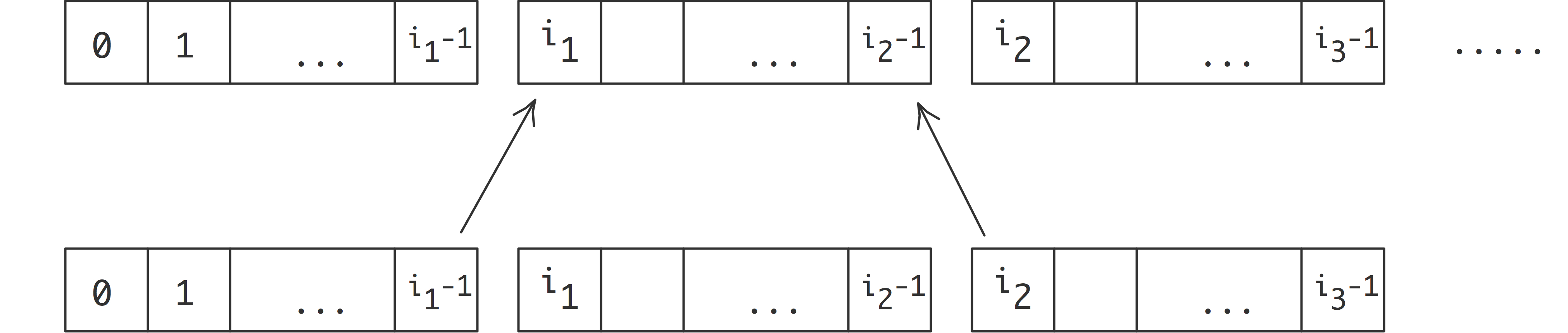FIGURE 4.2: Three point averaging in parallel, case of edge points

(figure  4.2  ). There is a similar story with the last index that $p$ tries to compute: that involves a value that is only present on $p+1$.

You see that there is a need for processor-to-processor, or technically point-to-point  , information exchange. MPI realizes this through matched send and receive calls:

• One process does a send to a specific other process;
• the other process does a specific receive from that source.

We will now discuss the send and receive routines in detail.

### 4.1.1 Example: ping-pong

crumb trail: > mpi-ptp > Blocking point-to-point operations > Example: ping-pong

A simple scenario for information exchange between just two processes is the ping-pong : process A sends data to process B, which sends data back to A. This is not an operation that is particularly relevant to applications, although it is often used as a benchmark. Here we discuss it for to explain basic ideas.

This means that process A executes the code

MPI_Send( /* to: */ B ..... );
MPI_Recv( /* from: */ B ... );

while process B executes
MPI_Recv( /* from: */ A ... );
MPI_Send( /* to: */ A ..... );

Since we are programming in SPMD mode, this means our program looks like:
if ( /* I am process A */ ) {
MPI_Send( /* to: */ B ..... );
MPI_Recv( /* from: */ B ... );
} else if ( /* I am process B */ ) {
MPI_Recv( /* from: */ A ... );
MPI_Send( /* to: */ A ..... );
}


Remark The structure of the send and receive calls shows the symmetric nature of MPI: every target process is reached with the same send call, no matter whether it's running on the same multicore chip as the sender, or on a computational node halfway across the machine room, taking several network hops to reach. Of course, any self-respecting MPI implementation optimizes for the case where sender and receiver have access to the same shared memory. This means that a send/recv pair is realized as a copy operation from the sender buffer to the receiver buffer, rather than a network transfer.
End of remark

### 4.1.2 Send call

crumb trail: > mpi-ptp > Blocking point-to-point operations > Send call

The blocking send command is MPI_Send  . Example:

// sendandrecv.c
double send_data = 1.;
MPI_Send
( /* send buffer/count/type: */ &send_data,1,MPI_DOUBLE,
/* to: */ receiver, /* tag: */ 0,
/* communicator: */ comm);


The send call has the following elements.

Buffer
The send buffer is described by a trio of buffer/count/datatype. See section  3.2.4 for discussion.

Target
The \indextermbusdef{messsage}{target} is an explicit process rank to send to. This rank is a number from zero up to the result of MPI_Comm_size  . It is allowed for a process to send to itself, but this may lead to a runtime deadlock ; see section  4.1.4 for discussion. The value MPI_PROC_NULL is allowed: using that as a target causes no message to be sent or received.

Tag
Next, a message can have a tag Many applications have each sender send only one message at a time to a given receiver. For the case where there are multiple simultaneous messages between the same sender / receiver pair, the tag can be used to disambiguate between the messages.

Often, a tag value of zero is safe to use. Indeed, OO interfaces to MPI typically have the tag as an optional parameter with value zero. If you do use tag values, you can use the key MPI_TAG_UB to query what the maximum value is that can be used; see section  15.1.2  .

Communicator
Finally, in common with the vast majority of MPI calls, there is a communicator argument that provides a context for the send transaction. In order to match a send and receive operation, they need to be in the same communicator.

MPL note MPL uses a default value for the tag, and it can deduce the type of the buffer. Sending a scalar becomes:

// sendscalar.cxx
if (comm_world.rank()==0) {
double pi=3.14;
comm_world.send(pi, 1);  // send to rank 1
cout << "sent: " << pi << '\n';
} else if (comm_world.rank()==1) {
double pi=0;
comm_world.recv(pi, 0);  // receive from rank 0
cout << "got : " << pi << '\n';
}


MPL note MPL can send static array s without further layout specification:

// sendarray.cxx
double v;
comm_world.send(v, 1);  // send to rank 1
comm_world.recv(v, 0);  // receive from rank 0


Sending vectors uses a general mechanism:

// sendbuffer.cxx
std::vector<double> v(8);
mpl::contiguous_layout<double> v_layout(v.size());
comm_world.send(v.data(), v_layout, 1);  // send to rank 1
comm_world.recv(v.data(), v_layout, 0);  // receive from rank 0


MPL note Noncontiguous iteratable objects can be send with a iterator_layout:

std::list<int> v(20, 0);
mpl::iterator_layout<int> l(v.begin(), v.end());
comm_world.recv(&(*v.begin()), l, 0);


crumb trail: > mpi-ptp > Blocking point-to-point operations > Receive call

The basic blocking receive command is MPI_Recv  .

An example:

double recv_data;
MPI_Recv
( /* recv buffer/count/type: */ &recv_data,1,MPI_DOUBLE,
/* from: */ sender, /* tag: */ 0,
/* communicator: */ comm,
/* recv status: */ MPI_STATUS_IGNORE);


This is similar in structure to the send call, with some exceptions.

Buffer
The receive buffer has the same buffer/count/data parameters as the send call. However, the count argument here indicates the size of the buffer, rather than the actual length of a message. This sets an upper bound on the length of the incoming message.

• For receiving messages with unknown length, use MPI_Probe ; section  4.4.1  .
• A message longer than the buffer size will give an overflow error, either returning an error, or ending your program; see section  15.2.2  .
The length of the received message can be determined from the status object; see section  4.3 for more detail.

Source
Mirroring the target argument of the MPI_Send call, MPI_Recv has a \indextermbusdef{message}{source} argument. This can be either a specific rank, or it can be the MPI_ANY_SOURCE wildcard. In the latter case, the actual source can be determined after the message has been received; see section  4.3  . A source value of MPI_PROC_NULL is also allowed, which makes the receive succeed immediately with no data received.

MPL note The constant mpl:: any_source equals MPI_ANY_SOURCE (by constexpr ). End of MPL note

Tag
Similar to the messsage source, the message tag of a receive call can be a specific value or a wildcard, in this case MPI_ANY_TAG  .

Python note Python calls sensible use a default tag=0  , but you can specify your own tag value. On the receive call, the tag wildcard is MPI.ANY_TAG  .

Communicator
The communicator argument almost goes without remarking.

Status
The MPI_Recv command has one parameter that the send call lacks: the MPI_Status object, describing the message status  . This gives information about the message received, for instance if you used wildcards for source or tag. See section  4.3 for more about the status object.

Remark If you're not interested in the status, as is the case in many examples in this book, you can specify the constant MPI_STATUS_IGNORE  . Note that the signature of MPI_Recv lists the status parameter as output'; this direction' of the parameter of course only applies if you do not specify this constant.
End of remark

Exercise Implement the ping-pong program. Add a timer using MPI_Wtime  . For the status argument of the receive call, use MPI_STATUS_IGNORE  .

• Run multiple ping-pongs (say a thousand) and put the timer around the loop. The first run may take longer; try to discard it.
• Run your code with the two communicating processes first on the same node, then on different nodes. Do you see a difference?
• Then modify the program to use longer messages. How does the timing increase with message size?
For bonus points, can you do a regression to determine $\alpha,\beta$? (There is a skeleton code pingpong in the repository)
End of exercise

Exercise Take your pingpong program and modify it to let half the processors be source and the other half the targets. Does the pingpong time increase? Does the observed behavior depend on how you choose the two sets?
End of exercise

### 4.1.4 Problems with blocking communication

You may be tempted to think that the send call puts the data somewhere in the network, and the sending code can progress after this call, as in figure  4.3  , left.

\leavevmode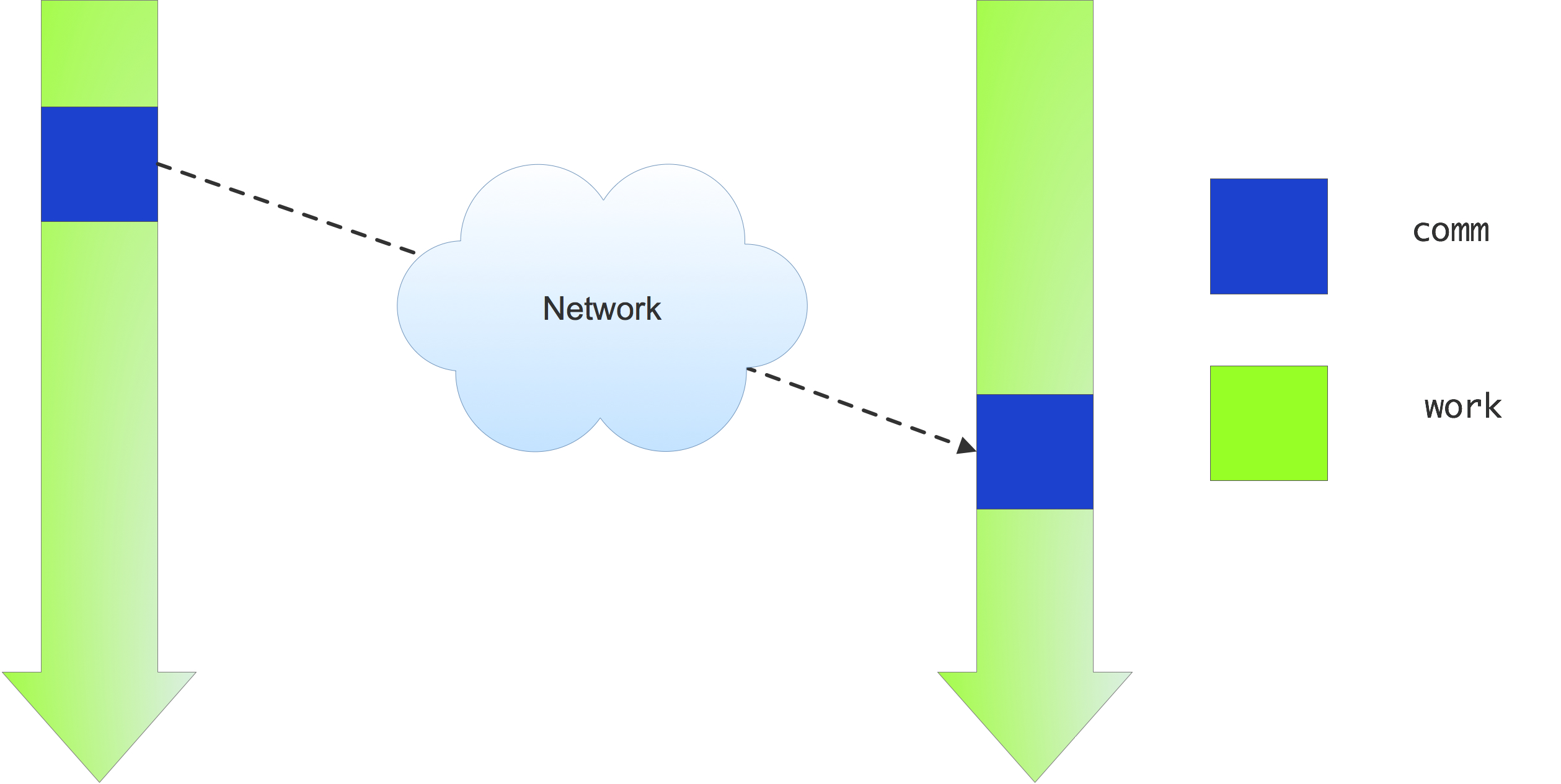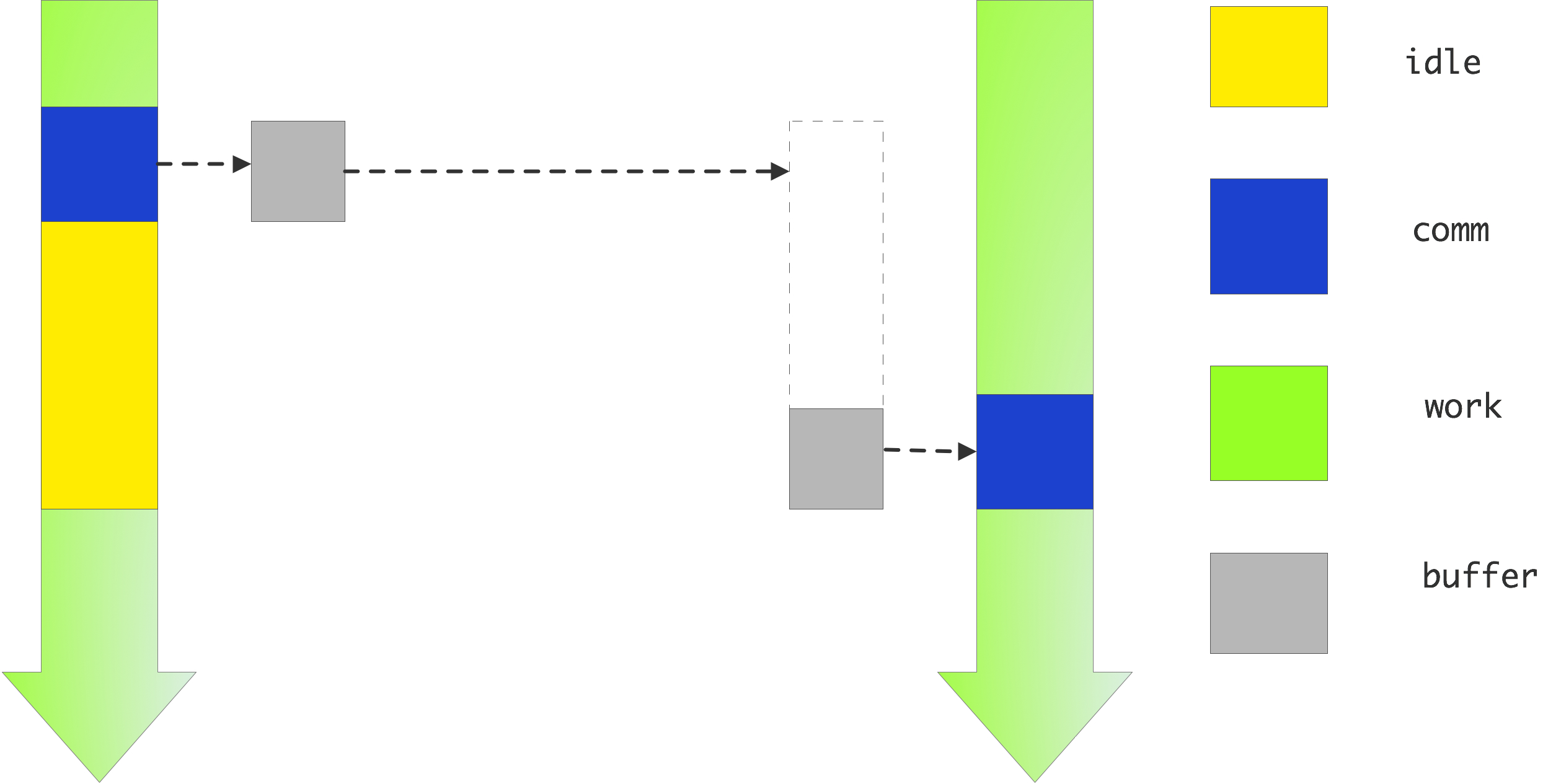FIGURE 4.3: Illustration of an ideal (left) and actual (right) send-receive interaction

But this ideal scenario is not realistic: it assumes that somewhere in the network there is buffer capacity for all messages that are in transit. This is not the case: data resides on the sender, and the sending call blocks, until the receiver has received all of it. (There is a exception for small messages, as explained in the next section.)

The use of MPI_Send and MPI_Recv is known as blocking communication : when your code reaches a send or receive call, it blocks until the call is succesfully completed. Technically, blocking operations are called non-local depends on factors that are not local to the process. See section  5.4  .

Suppose two process need to exchange data, and consider the following pseudo-code, which purports to exchange data between processes 0 and 1:

other = 1-mytid; /* if I am 0, other is 1; and vice versa */
send(target=other);

Imagine that the two processes execute this code. They both issue the send call\ldots\ and then can't go on, because they are both waiting for the other to issue the send call corresponding to their receive call. This is known as deadlock  .

#### 4.1.4.2 Eager vs rendezvous protocol

Messages can be sent using (at least) two different protocol s:

1. Rendezvous protocol, and
2. Eager protocol.
The rendezvous protocol is the most general. Sending a message takes several steps:
1. the sender sends a header, typically containing the message envelope : metadata describing the message;
3. the sender sends the actual data.
The purpose of this is to to prepare the receiver buffer space for large messages. However, it implies that the sender has to wait for some return message from the receiver, making the behavior a synchronous message  .

For the eager protocol, consider the example:

other = 1-mytid; /* if I am 0, other is 1; and vice versa */
send(target=other);

With a synchronous protocol you should get deadlock, since the send calls will be waiting for the receive operation to be posted.

In practice, however, this code will often work. The reason is that MPI implementations sometimes send small messages regardless of whether the receive has been posted. This is known as an eager send  , and it relies on the availability of some amount of available buffer space. The size under which this behavior is used is sometimes referred to as the eager limit  .

To illustrate eager and blocking behavior in MPI_Send  , consider an example where we send gradually larger messages. From the screen output you can see what the largest message was that fell under the eager limit; after that the code hangs because of a deadlock.

// sendblock.c
other = 1-procno;
/* loop over increasingly large messages */
for (int size=1; size<2000000000; size*=10) {
sendbuf = (int*) malloc(size*sizeof(int));
recvbuf = (int*) malloc(size*sizeof(int));
if (!sendbuf || !recvbuf) {
printf("Out of memory\n"); MPI_Abort(comm,1);
}
MPI_Send(sendbuf,size,MPI_INT,other,0,comm);
MPI_Recv(recvbuf,size,MPI_INT,other,0,comm,&status);
/* If control reaches this point, the send call
did not block. If the send call blocks,
we do not reach this point, and the program will hang.
*/
if (procno==0)
printf("Send did not block for size %d\n",size);
free(sendbuf); free(recvbuf);
}

!! sendblock.F90
other = 1-mytid
size = 1
do
allocate(sendbuf(size)); allocate(recvbuf(size))
print *,size
call MPI_Send(sendbuf,size,MPI_INTEGER,other,0,comm,err)
call MPI_Recv(recvbuf,size,MPI_INTEGER,other,0,comm,status,err)
if (mytid==0) then
print *,"MPI_Send did not block for size",size
end if
deallocate(sendbuf); deallocate(recvbuf)
size = size*10
if (size>2000000000) goto 20
end do
20   continue

## sendblock.py
size = 1
while size<2000000000:
sendbuf = np.empty(size, dtype=int)
recvbuf = np.empty(size, dtype=int)
comm.Send(sendbuf,dest=other)
comm.Recv(sendbuf,source=other)
if procid<other:
print("Send did not block for",size)
size *= 10


If you want a code to exhibit the same blocking behavior for all message sizes, you force the send call to be blocking by using MPI_Ssend  , which has the same calling sequence as MPI_Send  , but which does not allow eager sends.

// ssendblock.c
other = 1-procno;
sendbuf = (int*) malloc(sizeof(int));
recvbuf = (int*) malloc(sizeof(int));
size = 1;
MPI_Ssend(sendbuf,size,MPI_INT,other,0,comm);
MPI_Recv(recvbuf,size,MPI_INT,other,0,comm,&status);
printf("This statement is not reached\n");

Formally you can describe deadlock as follows. Draw up a graph where every process is a node, and draw a directed arc from process A to B if A is waiting for B. There is deadlock if this directed graph has a loop.

The solution to the deadlock in the above example is to first do the send from 0 to 1, and then from 1 to 0 (or the other way around). So the code would look like:

if ( /* I am processor 0 */ ) {
send(target=other);
} else {
send(target=other);
}


Eager sends also influences non-blocking The wait call after a non-blocking send will return immediately, regardless any receive call, if the message is under the eager limit: \csnippetwithoutput{eagerisend}{code/mpi/c}{eageri}

The eager limit is implementation-specific. For instance, for Intel MPI there is a variable I_MPI_EAGER_THRESHOLD (old versions) or I_MPI_SHM_EAGER_THRESHOLD ; for mvapich2 it is MV2_IBA_EAGER_THRESHOLD  , and for OpenMPI the --mca options btl_openib_eager_limit and btl_openib_rndv_eager_limit  .

#### 4.1.4.3 Serialization

There is a second, even more subtle problem with blocking communication. Consider the scenario where every processor needs to pass data to its successor, that is, the processor with the next higher rank. The basic idea would be to first send to your successor, then receive from your predecessor. Since the last processor does not have a successor it skips the send, and likewise the first processor skips the receive. The pseudo-code looks like:

successor = mytid+1; predecessor = mytid-1;
if ( /* I am not the last processor */ )
send(target=successor);
if ( /* I am not the first processor */ )


Exercise (Classroom exercise) Each student holds a piece of paper in the right hand -- keep your left hand behind your back -- and we want to execute:

1. Give the paper to your right neighbor;
2. Accept the paper from your left neighbor.
Including boundary conditions for first and last process, that becomes the following program:
1. If you are not the rightmost student, turn to the right and give the paper to your right neighbor.
2. If you are not the leftmost student, turn to your left and accept the paper from your left neighbor.

End of exercise

This code does not deadlock. All processors but the last one block on the send call, but the last processor executes the receive call. Thus, the processor before the last one can do its send, and subsequently continue to its receive, which enables another send, et cetera.

In one way this code does what you intended to do: it will terminate (instead of hanging forever on a deadlock) and exchange data the right way. However, the execution now suffers from unexpected serialization : only one processor is active at any time, so what should have been a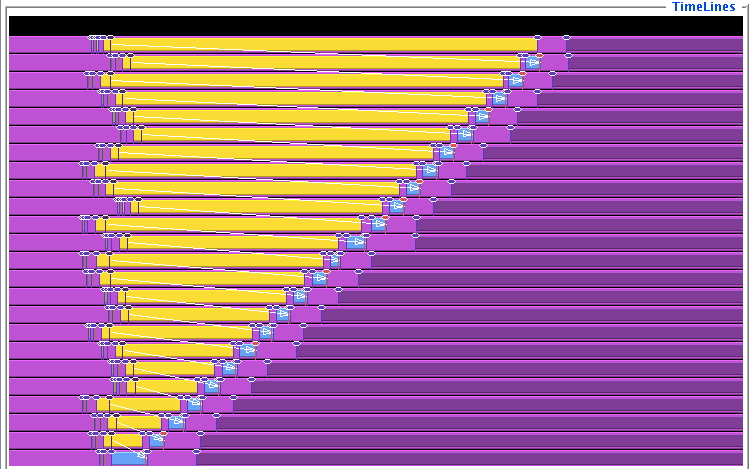FIGURE 4.4: Trace of a simple send-recv code

parallel operation becomes a sequential one. This is illustrated in figure  4.4  .

Exercise Implement the above algorithm using MPI_Send and MPI_Recv calls. Run the code, and use TAU to reproduce the trace output of figure  4.4  . If you don't have TAU, can you show this serialization behavior using timings, for instance running it on an increasing number of processes? (There is a skeleton code rightsend in the repository)
End of exercise

It is possible to orchestrate your processes to get an efficient and deadlock-free execution, but doing so is a bit cumbersome.

Exercise The above solution treated every processor equally. Can you come up with a solution that uses blocking sends and receives, but does not suffer from the serialization behavior?
End of exercise

There are better solutions which we will explore in the next section.

crumb trail: > mpi-ptp > Blocking point-to-point operations > Bucket brigade

The problem with the previous exercise was that an operation that was conceptually parallel became serial in execution. On the other hand, sometimes the operation is actually serial in nature. One example is the bucket brigade operation, where a piece of data is successively passed down a sequence of processors.

Exercise Take the code of exercise  4.4 and modify it so that the data from process zero gets propagated to every process. Specifically, compute all partial sums $\sum_{i=0}^pi^2$: \begin{equation} \begin{cases} x_0 = 1&\hbox{on process zero}\\ x_p = x_{p-1}+(p+1)^2 & \hbox{on process $p$}\\ \end{cases} \end{equation} Use MPI_Send and MPI_Recv ; make sure to get the order right.

Food for thought: all quantities involved here are integers. Is it a good idea to use the integer datatype here?

(There is a skeleton code bucketblock in the repository)
End of exercise

Remark There is an MPI_Scan routine (section  3.4  ) that performs the same computation, but computationally more efficiently. Thus, this exercise only serves to illustrate the principle.
End of remark

### 4.1.6 Pairwise exchange

crumb trail: > mpi-ptp > Blocking point-to-point operations > Pairwise exchange

Above you saw that with blocking sends the precise ordering of the send and receive calls is crucial. Use the wrong ordering and you get either deadlock, or something that is not efficient at all in parallel. MPI has a way out of this problem that is sufficient for many purposes: the combined send/recv call MPI_Sendrecv  .

The sendrecv call works great if every process is paired with precisely one sender and one receiver. You would then write

sendrecv( ....from... ...to... );

with the right choice of source and destination. For instance, to send data to your right neighbor:
MPI_Comm_rank(comm,&procno);
MPI_Sendrecv( ....
/* from: */ procno-1
... ...
/* to:   */ procno+1
... );

This scheme is correct for all processes but the first and last. In order to use the sendrecv call on these processes, we use MPI_PROC_NULL for the non-existing processes that the endpoints communicate with.
MPI_Comm_rank( .... &procno );
if ( /* I am not the first processor */ )
predecessor = procno-1;
else
predecessor = MPI_PROC_NULL;
if ( /* I am not the last processor */ )
successor = procno+1;
else
successor = MPI_PROC_NULL;
sendrecv(from=predecessor,to=successor);

where the sendrecv call is executed by all processors.

All processors but the last one send to their neighbor; the target value of MPI_PROC_NULL for the last processor means a send to the null processor': no actual send is done.

Likewise, receiving from MPI_PROC_NULL succeeds without altering the receive buffer. The corresponding MPI_Status object has source MPI_PROC_NULL  , tag MPI_ANY_TAG  , and count zero.

Remark The MPI_Sendrecv can inter-operate with the normal send and receive calls, both blocking and non-blocking. Thus it would also be possible to replace the MPI_Sendrecv calls at the end points by simple sends or receives.
End of remark

MPL note The send-recv call in MPL has the same possibilities for specifying the send and receive buffer as the separate send and recv calls: scalar, layout, iterator. However, out of the nine conceivably possible routine signatures, only the versions are available where the send and receive buffer are specified the same way. Also, the send and receive tag need to be specified; they do not have default values.

// sendrecv.cxx
mpl::tag t0(0);
comm_world.sendrecv
( mydata,sendto,t0,
leftdata,recvfrom,t0 );

End of MPL note

Exercise Revisit exercise  4.1.4.3 and solve it using MPI_Sendrecv  .

If you have TAU installed, make a trace. Does it look different from the serialized send/recv code? If you don't have TAU, run your code with different numbers of processes and show that the runtime is essentially constant.
End of exercise

This call makes it easy to exchange data between two processors: both specify the other as both target and source. However, there need not be any such relation between target and source: it is possible to receive from a predecessor in some ordering, and send to a successor in that ordering; see figure  4.5  .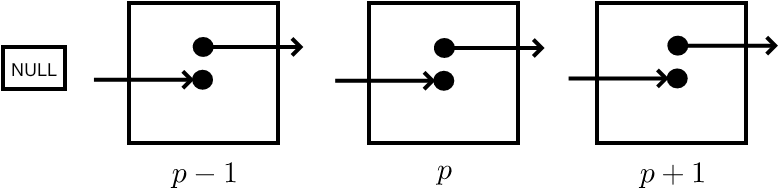FIGURE 4.5: An MPI Sendrecv callFIGURE 4.6: Two steps of send/recv to do a three-point combination

.

For the above three-point combination scheme you need to move data both left right, so you need two MPI_Sendrecv calls; see figure  4.6  .

Exercise Implement the above three-point combination scheme using MPI_Sendrecv ; every processor only has a single number to send to its neighbor. (There is a skeleton code sendrecv in the repository)
End of exercise

Hints for this exercise:

• Each process does one send and one receive; if a process needs to skip one or the other, you can specify MPI_PROC_NULL as the other process in the send or receive specification. In that case the corresponding action is not taken.
• As with the simple send/recv calls, processes have to match up: if process $p$ specifies $p'$ as the destination of the send part of the call, $p'$ needs to specify $p$ as the source of the recv part.

The following exercise lets you implement a sorting algorithm with the send-receive call\footnote {There is an MPI\_Compare\_and\_swap call. Do not use that.}.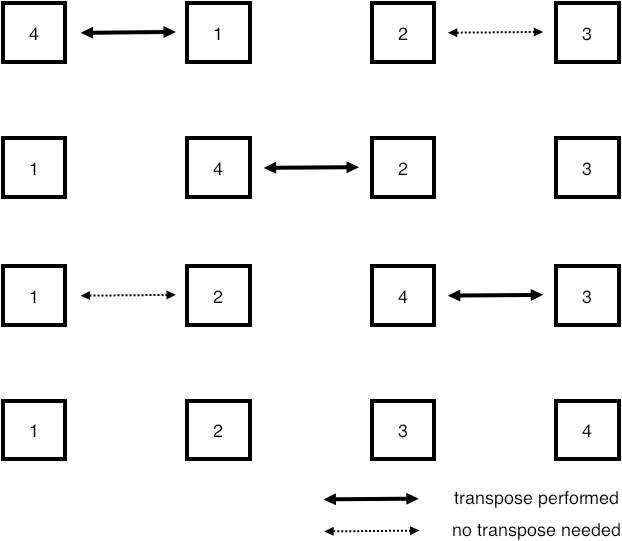FIGURE 4.7: Odd-even transposition sort on 4 elements.

Exercise A very simple sorting algorithm is swap sort or odd-even transposition sort : pairs of processors compare data, and if necessary exchange. The elementary step is called a compare-and-swap : in a pair of processors each sends their data to the other; one keeps the minimum values, and the other the maximum. For simplicity, in this exercise we give each processor just a single number.

The transposition sort algorithm is split in even and odd stages, where in the even stage processors $2i$ and $2i+1$ compare and swap data, and in the odd stage processors $2i+1$ and $2i+2$ compare and swap. You need to repeat this $P/2$ times, where $P$ is the number of processors; see figure  4.7  .

Implement this algorithm using MPI_Sendrecv  . (Use MPI_PROC_NULL for the edge cases if needed.) Use a gather call to print the global state of the distributed array at the beginning and end of the sorting process.
End of exercise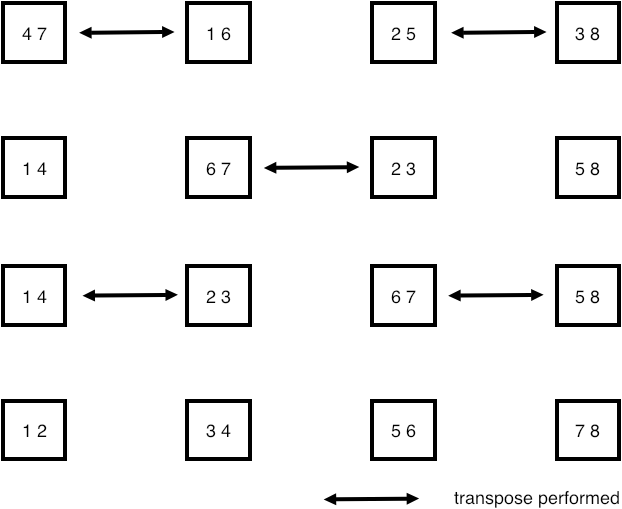FIGURE 4.8: Odd-even transposition sort on 4 processes, holding 2 elements each.

Remark It is not possible to use MPI_IN_PLACE for the buffers, as in section  3.3.2  . Instead, the routine MPI_Sendrecv_replace has only one buffer, used as both send and receive buffer. Of course, this requires the send and receive messages to fit in that one buffer.
End of remark

Exercise Extend this exercise to the case where each process hold an equal number of elements, more than 1. Consider figure  4.8 for inspiration. Is it coincidence that the algorithm takes the same number of steps as in the single scalar case?
End of exercise

\begin{mpifournote} {Non-blocking/persistent sendrecv} There are non-blocking and persistent versions of MPI_Sendrecv : MPI_Isendrecv  , MPI_Sendrecv_init  , MPI_Isendrecv_replace  , MPI_Sendrecv_replace_init  . \end{mpifournote}

## 4.2 Nonblocking point-to-point operations

crumb trail: > mpi-ptp > Nonblocking point-to-point operations

The structure of communication is often a reflection of the structure of the operation. With some regular applications we also get a regular communication pattern. Consider again the above operation: \begin{equation} y_i=x_{i-1}+x_i+x_{i+1}\colon i=1,…,N-1 \end{equation} Doing this in parallel induces communication, as pictured in figure  4.1  .

We note:

• The data is one-dimensional, and we have a linear ordering of the processors.
• The operation involves neighboring data points, and we communicate with neighboring processors.

Above you saw how you can use information exchange between pairs of processors

• using MPI_Send and MPI_Recv  , if you are careful; or
• using MPI_Sendrecv  , as long as there is indeed some sort of pairing of processors.
However, there are circumstances where it is not possible, not efficient, or simply not convenient, to have such a deterministic setup of the send and receive calls.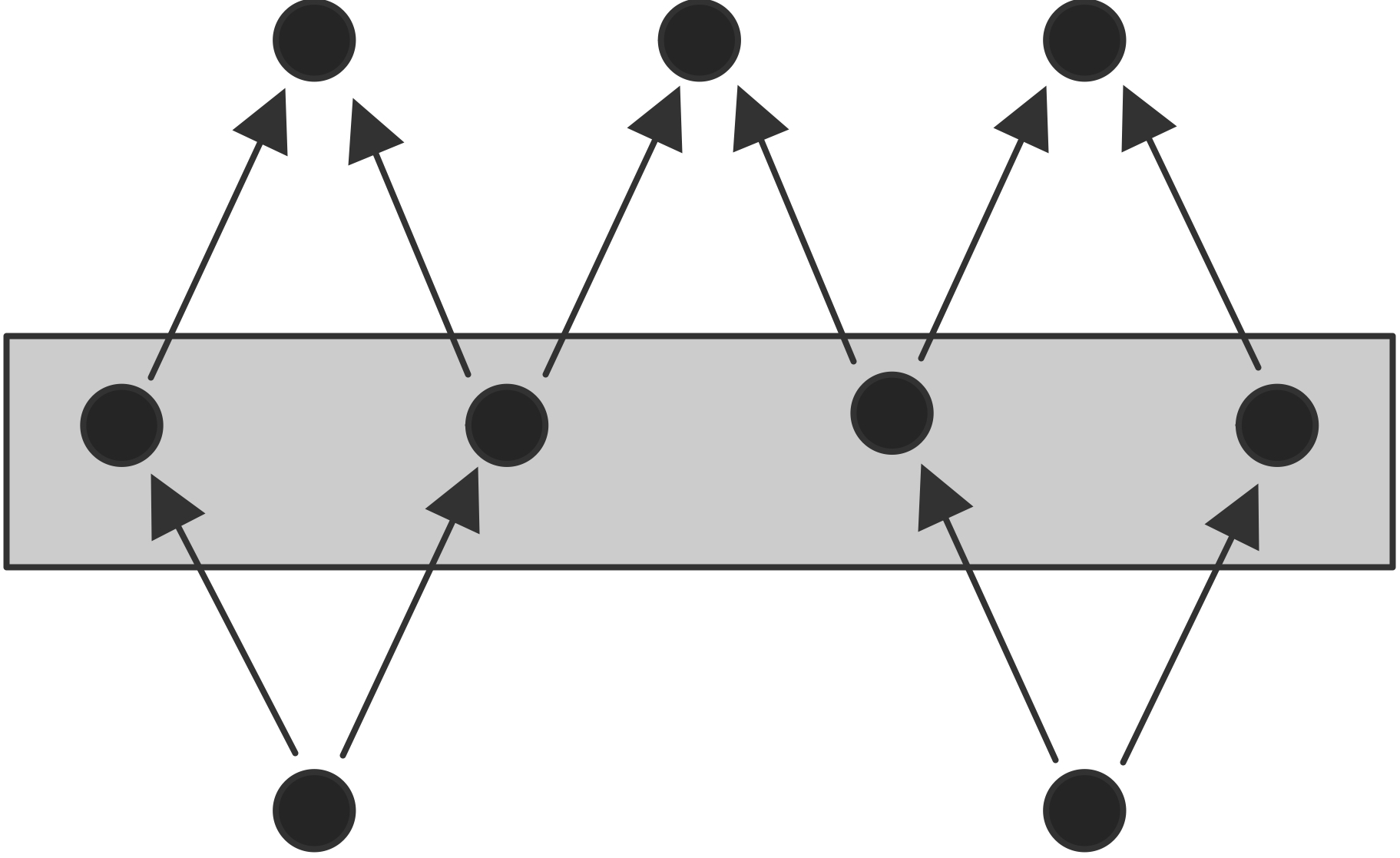FIGURE 4.9: Processors with unbalanced send/receive patterns

Figure  4.9 illustrates such a case, where processors are organized in a general graph pattern. Here, the numbers of sends and receive of a processor do not need to match.

In such cases, one wants a possibility to state these are the expected incoming messages', without having to wait for them in sequence. Likewise, one wants to declare the outgoing messages without having to do them in any particular sequence. Imposing any sequence on the sends and receives is likely to run into the serialization behavior observed above, or at least be inefficient.

### 4.2.1 Nonblocking send and receive calls

In the previous section you saw that blocking communication makes programming tricky if you want to avoid deadlock and performance problems. The main advantage of these routines is that you have full control about where the data is: if the send call returns the data has been successfully received, and the send buffer can be used for other purposes or de-allocated.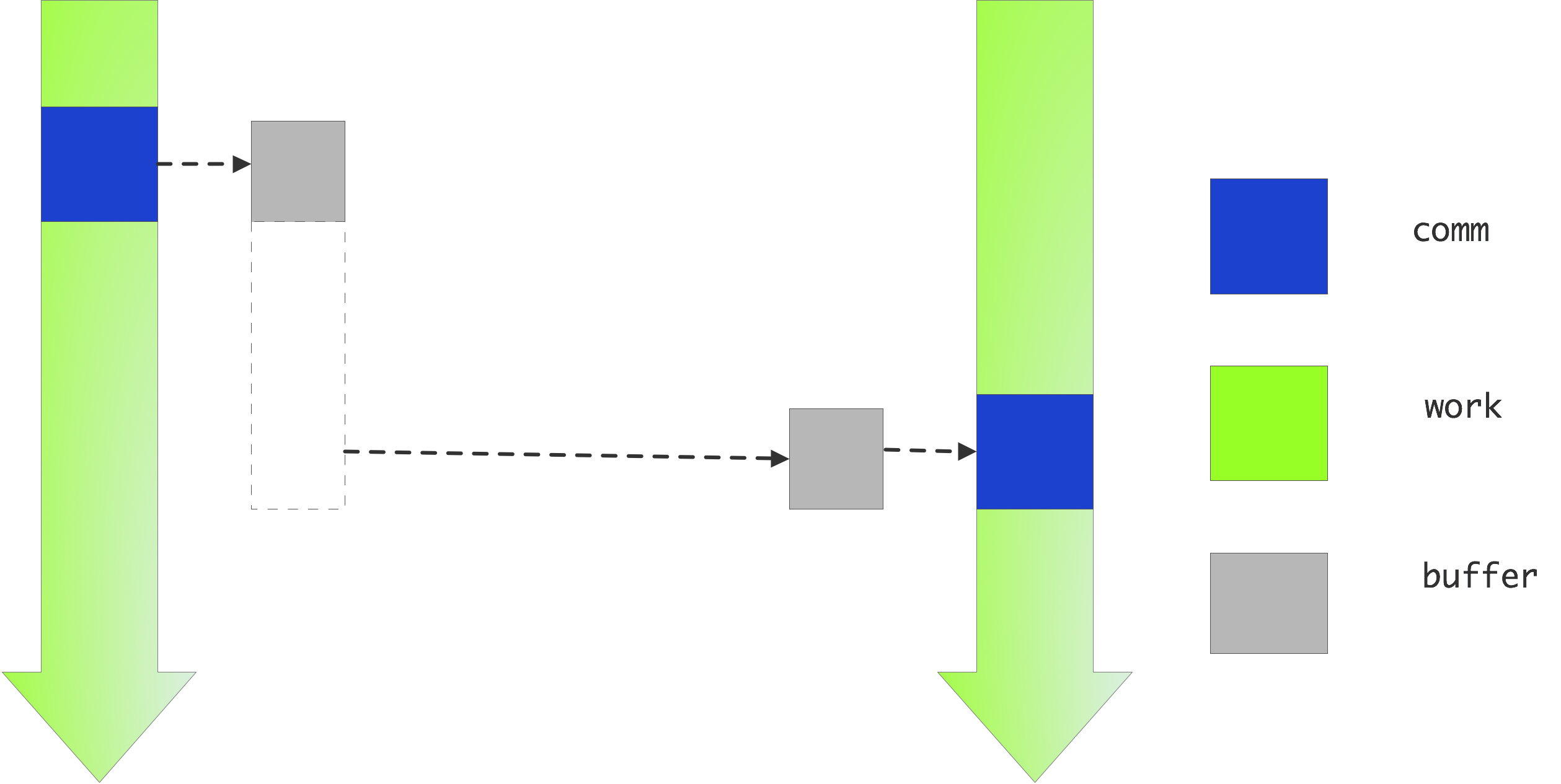FIGURE 4.10: Nonblocking send

By contrast, the nonblocking calls MPI_Isend and MPI_Irecv (where the I' stands for  immediate ' or  incomplete '  ) do not wait for their counterpart: in effect they tell the runtime system here is some data and please send it as follows' or here is some buffer space, and expect such-and-such data to come'. This is illustrated in figure  4.10  .

// isendandirecv.c
double send_data = 1.;
MPI_Request request;
MPI_Isend
( /* send buffer/count/type: */ &send_data,1,MPI_DOUBLE,
/* to: */ receiver, /* tag: */ 0,
/* communicator: */ comm,
/* request: */ &request);
MPI_Wait(&request,MPI_STATUS_IGNORE);

double recv_data;
MPI_Request request;
MPI_Irecv
( /* recv buffer/count/type: */ &recv_data,1,MPI_DOUBLE,
/* from: */ sender, /* tag: */ 0,
/* communicator: */ comm,
/* request: */ &request);
MPI_Wait(&request,MPI_STATUS_IGNORE);

Issuing the MPI_Isend  / MPI_Irecv call is sometimes referred to as posting a send/receive.

### 4.2.2 Request completion: wait calls

From the definition of MPI_Isend  / MPI_Irecv  , you seen that nonblocking routine yields an MPI_Request object. This request can then be used to query whether the operation has concluded. You may also notice that the MPI_Irecv routine does not yield an MPI_Status object. This makes sense: the status object describes the actually received data, and at the completion of the MPI_Irecv call there is no received data yet.

Waiting for the request is done with a number of routines. We first consider MPI_Wait  . It takes the request as input, and gives an MPI_Status as output. If you don't need the status object, you can pass MPI_STATUS_IGNORE  .

// hangwait.c
if (procno==sender) {
for (int p=0; p<nprocs-1; p++) {
double send = 1.;
MPI_Send( &send,1,MPI_DOUBLE,p,0,comm);
}
} else {
double recv=0.;
MPI_Request request;
MPI_Irecv( &recv,1,MPI_DOUBLE,sender,0,comm,&request);
do_some_work();
MPI_Wait(&request,MPI_STATUS_IGNORE);
}


(Note that this example uses a mix of blocking and non-blocking operations: a blocking send is paired with a non-blocking receive.)

The request is passed by reference, so that the wait routine can free it:

• The wait call deallocates the request object, and
• sets the value of the variable to MPI_REQUEST_NULL  .
(See section  4.2.4 for details.)

MPL note Nonblocking routines have an irequest as function result. Note: not a parameter passed by reference, as in the C interface. The various wait calls are methods of the irequest class.

double recv_data;
mpl::irequest recv_request =
comm_world.irecv( recv_data,sender );
recv_request.wait();

You can not default-construct the request variable:
// DOES NOT COMPILE:
mpl::irequest recv_request;
recv_request = comm.irecv( ... );

This means that the normal sequence of first declaring, and then filling in, the request variable is not possible.

The wait call always returns a status object; not assigning it means that the destructor is called on it. End of MPL note

Now we discuss in some detail the various wait calls. These are blocking; for the nonblocking versions see section  4.2.3  .

#### 4.2.2.1 Wait for one request

MPI_Wait waits for a a single request. If you are indeed waiting for a single nonblocking communication to complete, this is the right routine. If you are waiting for multiple requests you could call this routine in a loop.

for (p=0; p<nrequests ; p++) // Not efficient!
MPI_Wait(&request[p],&(status[p]));


However, this would be inefficient if the first request is fulfilled much later than the others: your waiting process would have lots of idle time. In that case, use one of the following routines.

#### 4.2.2.2 Wait for all requests

MPI_Waitall allows you to wait for a number of requests, and it does not matter in what sequence they are satisfied. Using this routine is easier to code than the loop above, and it could be more efficient.

// irecvloop.c
MPI_Request requests =
(MPI_Request*) malloc( 2*nprocs*sizeof(MPI_Request) );
recv_buffers = (int*) malloc( nprocs*sizeof(int) );
send_buffers = (int*) malloc( nprocs*sizeof(int) );
for (int p=0; p<nprocs; p++) {
int
left_p  = (p-1+nprocs) % nprocs,
right_p = (p+1) % nprocs;
send_buffer[p] = nprocs-p;
MPI_Isend(sendbuffer+p,1,MPI_INT, right_p,0, requests+2*p);
MPI_Irecv(recvbuffer+p,1,MPI_INT, left_p,0, requests+2*p+1);
}
/* your useful code here */
MPI_Waitall(2*nprocs,requests,MPI_STATUSES_IGNORE);


The output argument is an array or MPI_Status object. If you don't need the status objects, you can pass MPI_STATUSES_IGNORE  .

As an illustration, we realize exercise  4.4  , and its trace in figure  4.4  , with non-blocking execution and MPI_Waitall  . Figure  4.11 shows the trace of this variant of the code.FIGURE 4.11: A trace of a nonblocking send between neighboring processors

Exercise Revisit exercise  4.1.5 and consider replacing the blocking calls by nonblocking ones. How far apart can you put the MPI_Isend  / MPI_Irecv calls and the corresponding MPI_Wait s? (There is a skeleton code bucketpipenonblock in the repository)
End of exercise

Exercise Create two distributed arrays of positive integers. Take the set difference of the two: the first array needs to be transformed to remove from it those numbers that are in the second array.

How could you solve this with an MPI_Allgather call? Why is it not a good idea to do so? Solve this exercise instead with a circular bucket brigade algorithm. (There is a skeleton code setdiff in the repository)
End of exercise

Python note Non-blocking routines such as \indexmpishowp{MPI_Isend} return a request object. The \indexmpishowp{MPI_Wait} is a class method, not a method of the request object:

## irecvsingle.py
sendbuffer = np.empty( nprocs, dtype=int )
recvbuffer = np.empty( nprocs, dtype=int )

left_p = (procid-1) % nprocs
right_p = (procid+1) % nprocs
send_request = comm.Isend\
( sendbuffer[procid:procid+1],dest=left_p)
recv_request = comm.Irecv\
( sendbuffer[procid:procid+1],source=right_p)
MPI.Request.Wait(send_request)
MPI.Request.Wait(recv_request)


Python note An array of requests (for the waitall/some/any calls) is an ordinary Python list:

## irecvloop.py
requests = []
sendbuffer = np.empty( nprocs, dtype=int )
recvbuffer = np.empty( nprocs, dtype=int )

for p in range(nprocs):
left_p = (p-1) % nprocs
right_p = (p+1) % nprocs
requests.append( comm.Isend\
( sendbuffer[p:p+1],dest=left_p) )
requests.append( comm.Irecv\
( sendbuffer[p:p+1],source=right_p) )
MPI.Request.Waitall(requests)

The \indexmpishowp{MPI_Waitall} method is again a class method.

#### 4.2.2.3 Wait for any requests

The waitall' routine is good if you need all nonblocking communications to be finished before you can proceed with the rest of the program. However, sometimes it is possible to take action as each request is satisfied. In that case you could use MPI_Waitany and write:

for (p=0; p<nrequests; p++) {
MPI_Irecv(buffer+index, /* ... */, requests+index);
}
for (p=0; p<nrequests; p++) {
MPI_Waitany(nrequests,request_array,&index,&status);
// operate on buffer[index]
}


Note that this routine takes a single status argument, passed by reference, and not an array of statuses!

Fortran note The index parameter is the index in the array of requests, which is a Fortran array, so it uses 1-based indexing  .

!! irecvsource.F90
if (mytid==ntids-1) then
do p=1,ntids-1
print *,"post"
call MPI_Irecv(recv_buffer(p),1,MPI_INTEGER,p-1,0,comm,&
requests(p),err)
end do
do p=1,ntids-1
call MPI_Waitany(ntids-1,requests,index,MPI_STATUS_IGNORE,err)
write(*,'("Message from",i3,":",i5)') index,recv_buffer(index)
end do

!! waitnull.F90
Type(MPI_Request),dimension(:),allocatable :: requests
allocate(requests(ntids-1))
call MPI_Waitany(ntids-1,requests,index,MPI_STATUS_IGNORE)
if ( .not. requests(index)==MPI_REQUEST_NULL) then
print *,"This request should be null:",index

!! waitnull.F90
Type(MPI_Request),dimension(:),allocatable :: requests
allocate(requests(ntids-1))
call MPI_Waitany(ntids-1,requests,index,MPI_STATUS_IGNORE)
if ( .not. requests(index)==MPI_REQUEST_NULL) then
print *,"This request should be null:",index

End of Fortran note

MPL note Instead of an array of requests, use an irequest_pool object, which acts like a vector of requests, meaning that you can push onto it.

// irecvsource.cxx
mpl::irequest_pool recv_requests;
for (int p=0; p<nprocs-1; p++) {
recv_requests.push( comm_world.irecv( recv_buffer[p], p ) );
}


You can not declare a pool of a fixed size and assign elements. End of MPL note

MPL note The irequest_pool class has methods waitany, waitall, testany, testall, waitsome, testsome.

The any' methods return a std::pair , with \lstinline{false} meaning index==MPI_UNDEFINED meaning no more requests to be satisfied.

  auto [success,index] = recv_requests.waitany();
if (success) {
auto recv_status = recv_requests.get_status(index);


Same for testany  , then false means no requests test true. End of MPL note

MPL note

  auto [success,index] = recv_requests.waitany();
if (success) {
auto recv_status = recv_requests.get_status(index);

End of MPL note

#### 4.2.2.4 Polling with MPI Wait any

The MPI_Waitany routine can be used to implement polling : occasionally check for incoming messages while other work is going on. \csnippetwithoutput{waitforany}{examples/mpi/c}{irecvsource}

## irecvsource.py
if procid==nprocs-1:
requests = [ None ] * (nprocs-1)
for sender in range(nprocs-1):
# alternatively: requests = [ comm.Irecv(s) for s in .... ]
status = MPI.Status()
for sender in range(nprocs-1):
ind = MPI.Request.Waitany(requests,status=status)
if ind!=status.Get_source():
print("sender mismatch: %d vs %d" % (ind,status.Get_source()))
else:
mywait = random.randint(1,2*nprocs)
print("[%d] wait for %d seconds" % (procid,mywait))
time.sleep(mywait)
mydata = np.empty(1,dtype=int)
mydata = procid
comm.Send([mydata,MPI.INT],dest=nprocs-1)

Each process except for the root does a blocking send; the root posts MPI_Irecv from all other processors, then loops with MPI_Waitany until all requests have come in. Use MPI_SOURCE to test the index parameter of the wait call.

Note the MPI_STATUS_IGNORE parameter: we know everything about the incoming message, so we do not need to query a status object. Contrast this with the example in section  4.3.1  .

#### 4.2.2.5 Wait for some requests

Finally, MPI_Waitsome is very much like MPI_Waitany  , except that it returns multiple numbers, if multiple requests are satisfied. Now the status argument is an array of MPI_Status objects.

#### 4.2.2.6 Receive status of the wait calls

The MPI_Wait... routines have the MPI_Status objects as output. If you are not interested in the status information, you can use the values MPI_STATUS_IGNORE for MPI_Wait and MPI_Waitany  , or MPI_STATUSES_IGNORE for MPI_Waitall  , MPI_Waitsome  , MPI_Testall  , MPI_Testsome  .

Remark The routines that can return multiple statuses, can return the error condition MPI_ERR_IN_STATUS  , indicating that one of the statuses was in error. See section  4.3.3  .
End of remark

Exercise (There is a skeleton code isendirecv in the repository) Now use nonblocking send/receive routines to implement the three-point averaging operation \begin{equation} y_i=\bigl( x_{i-1}+x_i+x_{i+1} \bigr)/3\colon i=1,…,N-1 \end{equation} on a distributed array. There are two approaches to the first and last process:

1. you can use MPI_PROC_NULL for the missing' communications;
2. you can skip these communications altogether, but now you have to count the requests carefully.

End of exercise

#### 4.2.2.7 Latency hiding / overlapping communication and computation

There is a second motivation for the Isend/Irecv calls: if your hardware supports it, the communication can happen while your program can continue to do useful work:

// start nonblocking communication
MPI_Isend( ... ); MPI_Irecv( ... );
// do work that does not depend on incoming data
....
// wait for the Isend/Irecv calls to finish
MPI_Wait( ... );
// now do the work that absolutely needs the incoming data
....

This is known as overlapping computation and communication  , or \indextermbusdef{latency}{hiding}. See also asynchronous progress ; section  15.4  .

Unfortunately, a lot of this communication involves activity in user space, so the solution would have been to let it be handled by a separate thread. Until recently, processors were not efficient at doing such multi-threading, so true overlap stayed a promise for the future. Some network cards have support for this overlap, but it requires a nontrivial combination of hardware, firmware, and MPI implementation.

Exercise (There is a skeleton code isendirecvarray in the repository) Take your code of exercise  4.2.2.6 and modify it to use latency hiding. Operations that can be performed without needing data from neighbors should be performed in between the MPI_Isend  / MPI_Irecv calls and the corresponding MPI_Wait calls.
End of exercise

Remark You have now seen various send types: blocking, nonblocking, synchronous. Can a receiver see what kind of message was sent? Are different receive routines needed? The answer is that, on the receiving end, there is nothing to distinguish a nonblocking or synchronous message. The MPI_Recv call can match any of the send routines you have seen so far, and conversely a message sent with MPI_Send can be received by MPI_Irecv  .
End of remark

#### 4.2.2.8 Buffer issues in nonblocking communication

While the use of nonblocking routines prevents deadlock, it introduces problems of its own.

• With a blocking send call, you could repeatedly fill the send buffer and send it off.
double *buffer;
for ( ... p ... ) {
buffer = // fill in the data
MPI_Send( buffer, ... /* to: */ p );

• On the other hand, when a nonblocking send call returns, the actual send may not have been executed, so the send buffer may not be safe to overwrite. Similarly, when the recv call returns, you do not know for sure that the expected data is in it. Only after the corresponding wait call are you use that the buffer has been sent, or has received its contents.
• To send multiple messages with nonblocking calls you therefore have to allocate multiple buffers.
double **buffers;
for ( ... p ... ) {
buffers[p] = // fill in the data
MPI_Send( buffers[p], ... /* to: */ p );
}
MPI_Wait( /* the requests */ );


// irecvloop.c
MPI_Request requests =
(MPI_Request*) malloc( 2*nprocs*sizeof(MPI_Request) );
recv_buffers = (int*) malloc( nprocs*sizeof(int) );
send_buffers = (int*) malloc( nprocs*sizeof(int) );
for (int p=0; p<nprocs; p++) {
int
left_p  = (p-1+nprocs) % nprocs,
right_p = (p+1) % nprocs;
send_buffer[p] = nprocs-p;
MPI_Isend(sendbuffer+p,1,MPI_INT, right_p,0, requests+2*p);
MPI_Irecv(recvbuffer+p,1,MPI_INT, left_p,0, requests+2*p+1);
}
/* your useful code here */
MPI_Waitall(2*nprocs,requests,MPI_STATUSES_IGNORE);


### 4.2.3 Wait and test calls

crumb trail: > mpi-ptp > Nonblocking point-to-point operations > Wait and test calls

The MPI_Wait... routines are blocking. Thus, they are a good solution if the receiving process can not do anything until the data (or at least some data) is actually received. The MPI_Test... calls are themselves nonblocking: they test for whether one or more requests have been fullfilled, but otherwise immediately return. It is also a local operation : it does not force progress.

The MPI_Test call can be used in the manager-worker model: the manager process creates tasks, and sends them to whichever worker process has finished its work. (This uses a receive from MPI_ANY_SOURCE  , and a subsequent test on the MPI_SOURCE field of the receive status.) While waiting for the workers, the manager can do useful work too, which requires a periodic check on incoming message.

Pseudo-code:

while ( not done ) {
// create new inputs for a while
....
// see if anyone has finished
MPI_Test( .... &index, &flag );
if ( flag ) {
// receive processed data and send new
}


If the test is true, the request is deallocated and set to MPI_REQUEST_NULL  , or, in the case of an active persistent request (section  5.1  ), set to inactive.

Analogous to MPI_Wait  , MPI_Waitany  , MPI_Waitall  , MPI_Waitsome  , there are MPI_Test  , MPI_Testany  , MPI_Testall  , MPI_Testsome  .

Exercise Read section  Eijkhout:IntroHPC and give pseudo-code for the distributed sparse matrix-vector product using the above idiom for using MPI_Test... calls. Discuss the advantages and disadvantages of this approach. The answer is not going to be black and white: discuss when you expect which approach to be preferable.
End of exercise

crumb trail: > mpi-ptp > Nonblocking point-to-point operations > More about requests

Every nonblocking call allocates an MPI_Request object. Unlike MPI_Status  , an MPI_Request variable is not actually an object, but instead it is an (opaque) pointer. This meeans that when you call, for instance, MPI_Irecv  , MPI will allocate an actual request object, and return its address in the MPI_Request variable.

Correspondingly, calls to MPI_Wait or MPI_Test free this object, setting the handle to MPI_REQUEST_NULL  . (There is an exception for persistent communications where the request is only set to inactive'; section  5.1  .) Thus, it is wise to issue wait calls even if you know that the operation has succeeded. For instance, if all receive calls are concluded, you know that the corresponding send calls are finished and there is no strict need to wait for their requests. However, omitting the wait calls would lead to a memory leak  .

Another way around this is to call MPI_Request_free  , which sets the request variable to MPI_REQUEST_NULL  , and marks the object for deallocation after completion of the operation. Conceivably, one could issue a nonblocking call, and immediately call MPI_Request_free  , dispensing with any wait call. However, this makes it hard to know when the operation is concluded and when the buffer is safe to reuse  [Squyres:evilrequest]  .

You can inspect the status of a request without freeing the request object with MPI_Request_get_status  .

## 4.3 The Status object and wildcards

crumb trail: > mpi-ptp > The Status object and wildcards

In section  4.1.1 you saw that MPI_Recv has a status' argument of type MPI_Status that MPI_Send lacks. (The various MPI_Wait... routines also have a status argument; see section  4.2.1  .) Often you specify MPI_STATUS_IGNORE for this argument: commonly you know what data is coming in and where it is coming from.

However, in some circumstances the recipient may not know all details of a message when you make the receive call, so MPI has a way of querying the status of the message:

• If you are expecting multiple incoming messages, it may be most efficient to deal with them in the order in which they arrive. So, instead of waiting for a specific message, you would specify MPI_ANY_SOURCE or MPI_ANY_TAG in the description of the receive message. Now you have to be able to ask who did this message come from, and what is in it'.
• Maybe you know the sender of a message, but the amount of data is unknown. In that case you can overallocate your receive buffer, and after the message is received ask how big it was, or you can probe' an incoming message and allocate enough data when you find out how much data is being sent.

To do this, the receive call has a MPI_Status parameter. The MPI_Status object is a structure (in C a struct  , in F90 an array, in F2008 a derived type) with freely accessible members:

• MPI_SOURCE gives the source of the message; see section  4.3.1  .
• MPI_TAG gives the tag with which the message was received; see section  4.3.2  .
• MPI_ERROR gives the error status of the receive call; see section  4.3.3  .
• The number of items in the message can be deduced from the status object, not as a structure member, but through a function call to MPI_Get_count ; see section  4.3.4  .

Fortran note The mpi_f08 module turns many handles (such as communicators) from Fortran Integer s into Type s. Retrieving the integer from the type is usually done through the %val member, but for the status object this is more difficult. The routines MPI_Status_f2f08 and MPI_Status_f082f convert between these. (Remarkably, these routines are even available in C, where they operate on MPI_Fint  , MPI_F08_status arguments.) End of Fortran note

Python note The status object is explicitly created before being passed to the receive routine. It has the usual query method for the message count:

## pingpongbig.py
status = MPI.Status()
comm.Recv( rdata,source=0,status=status)
count = status.Get_count(MPI.DOUBLE)


(The count function without argument returns a result in bytes.)

However, unlike in C/F where the fields of the status object are directly accessible, Python has query methods for these too:

    status.Get_source()
status.Get_tag()
status.Get_elements()
status.Get_error()
status.Is_cancelled()

Should you need them, there are even Set variants of these.

MPL note The mpl::status_t object is created by the receive (or wait) call:

mpl::contiguous_layout<double> target_layout(count);
mpl::status_t recv_status =
comm_world.recv(target.data(),target_layout, the_other);
recv_count = recv_status.get_count<double>();

End of MPL note

### 4.3.1 Source

crumb trail: > mpi-ptp > The Status object and wildcards > Source

In some applications it makes sense that a message can come from one of a number of processes. In this case, it is possible to specify MPI_ANY_SOURCE as the source. To find out the source where the message actually came from, you would use the MPI_SOURCE field of the status object that is delivered by MPI_Recv or the MPI_Wait... call after an MPI_Irecv  .

MPI_Recv(recv_buffer+p,1,MPI_INT, MPI_ANY_SOURCE,0,comm,
&status);
sender = status.MPI_SOURCE;


There are various scenarios where receiving from any source' makes sense. One is that of the manager-worker model. The manager task would first send data to the worker tasks, then issues a blocking wait for the data of whichever process finishes first.

In Fortran2008 style, the source is a member of the \flstinline{Status} type.

!! anysource.F90
Type(MPI_Status)  :: status
allocate(recv_buffer(ntids-1))
do p=0,ntids-2
call MPI_Recv(recv_buffer(p+1),1,MPI_INTEGER,&
MPI_ANY_SOURCE,0,comm,status)
sender = status%MPI_SOURCE


In Fortran90 style, the source is an index in the \flstinline{Status} array.

!! anysource.F90
integer :: status(MPI_STATUS_SIZE)
allocate(recv_buffer(ntids-1))
do p=0,ntids-2
call MPI_Recv(recv_buffer(p+1),1,MPI_INTEGER,&
MPI_ANY_SOURCE,0,comm,status,err)
sender = status(MPI_SOURCE)


MPL note The status object can be queried:

int source = recv_status.source();

End of MPL note

### 4.3.2 Tag

crumb trail: > mpi-ptp > The Status object and wildcards > Tag

In some circumstances, a tag wildcard can be used on the receive operation: MPI_ANY_TAG  . The actual tag of a message can be retrieved as the MPI_TAG member in the status structure.

There are not many cases where this is needed.

• Messages from a single source, even non-blocking, are non-overtaking  . This means that messages can be distinguished by their order.
• Messages from multiple sources can be distinguished by the source field.
• Retrieving the message tag might be needed if information is encoded in it.
• The non-overtaking argument does not apply in the case of hybrid computing: two threads may send messages that do not have an MPI-imposed order. See the example in section  45.1  .

MPL note Tag are int or an enum typ:

    template<typename T >
tag_t (T t);
tag_t (int t);

Example:
// inttag.cxx
enum class tag : int { ping=1, pong=2 };
int pinger = 0, ponger = world.size()-1;
if (world.rank()==pinger) {
world.send(x, 1, tag::ping);
world.recv(x, 1, tag::pong);
} else if (world.rank()==ponger) {
world.recv(x, 0, tag::ping);
world.send(x, 0, tag::pong);
}

End of MPL note

MPL note MPL differs from other APIs in its treatment of tags: a tag is not directly an integer, but an object of class tag.

// sendrecv.cxx
mpl::tag t0(0);
comm_world.sendrecv
( mydata,sendto,t0,
leftdata,recvfrom,t0 );

The tag class has a couple of methods such as mpl:: tag::any\lstinline+()+ (for the MPI_ANY_TAG wildcard in receive calls) and mpl:: tag::up\lstinline+()+ (maximal tag, found from the MPI_TAG_UB attribute). End of MPL note

### 4.3.3 Error

crumb trail: > mpi-ptp > The Status object and wildcards > Error

For functions that return a single status, any error is returned as the function result. For a function returning multiple statuses, such as MPI_Waitall  , the presence of an error in one of the receives is indicated by a result of MPI_ERR_IN_STATUS  . Any errors during the receive operation can be found as the MPI_ERROR member of the status structure.

### 4.3.4 Count

crumb trail: > mpi-ptp > The Status object and wildcards > Count

If the amount of data received is not known a priori, the count can be found by MPI_Get_count :

// count.c
if (procid==0) {
int sendcount = (rand()>.5) ? N : N-1;
MPI_Send( buffer,sendcount,MPI_FLOAT,target,0,comm );
} else if (procid==target) {
MPI_Status status;
int recvcount;
MPI_Recv( buffer,N,MPI_FLOAT,0,0, comm, &status );
MPI_Get_count(&status,MPI_FLOAT,&recvcount);
}

\fsnippetwithoutput{mpigetcountf08}{examples/mpi/f08}{count}

This may be necessary since the count argument to MPI_Recv is the buffer size, not an indication of the actually received number of data items.

Remarks.

• Unlike the source and tag, the message count is not directly a member of the status structure.
• The count' returned is the number of elements of the specified datatype. If this is a derived type (section  6.3  ) this is not the same as the number of predefined datatype elements. For that, use MPI_Get_elements or MPI_Get_elements_x which returns the number of basic elements.

MPL note The get_count function is a method of the status object. The argument type is handled through templating:

// recvstatus.cxx
double pi=0;
auto s = comm_world.recv(pi, 0);  // receive from rank 0
int c = s.get_count<double>();
std::cout << "got : " << c << " scalar(s): " << pi << '\n';

End of MPL note

### 4.3.5 Example: receiving from any source

Consider an example where the last process receives from every other process. We could implement this as a loop

for (int p=0; p<nprocs-1; p++)
MPI_Recv( /* from source= */ p );

but this may incur idle time if the messages arrive out of order.

Instead, we use the MPI_ANY_SOURCE specifier to give a wildcard behavior to the receive call: using this value for the source' value means that we accept mesages from any source within the communicator, and messages are only matched by tag value. (Note that size and type of the receive buffer are not used for message matching!)

We then retrieve the actual source from the MPI_Status object through the MPI_SOURCE field.

// anysource.c
if (procno==nprocs-1) {
/*
* The last process receives from every other process
*/
int *recv_buffer;
recv_buffer = (int*) malloc((nprocs-1)*sizeof(int));

/*
* Messages can come in in any order, so use MPI_ANY_SOURCE
*/
MPI_Status status;
for (int p=0; p<nprocs-1; p++) {
err = MPI_Recv(recv_buffer+p,1,MPI_INT, MPI_ANY_SOURCE,0,comm,
&status); CHK(err);
int sender = status.MPI_SOURCE;
printf("Message from sender=%d: %d\n",
sender,recv_buffer[p]);
}
free(recv_buffer);
} else {
/*
* Each rank waits an unpredictable amount of time,
* then sends to the last process in line.
*/
float randomfraction = (rand() / (double)RAND_MAX);
int randomwait = (int) ( nprocs * randomfraction );
printf("process %d waits for %e/%d=%d\n",
procno,randomfraction,nprocs,randomwait);
sleep(randomwait);
err = MPI_Send(&randomwait,1,MPI_INT, nprocs-1,0,comm); CHK(err);
}

## anysource.py
rstatus = MPI.Status()
comm.Recv(rbuf,source=MPI.ANY_SOURCE,status=rstatus)
print("Message came from %d" % rstatus.Get_source())


The manager-worker model is a design patterns that offers an opportunity for inspecting the MPI_SOURCE field of the MPI_Status object describing the data that was received. All workers processes model their work by waitin a random amount of time, and the manager process accepts messages from any source.

// anysource.c
if (procno==nprocs-1) {
/*
* The last process receives from every other process
*/
int *recv_buffer;
recv_buffer = (int*) malloc((nprocs-1)*sizeof(int));

/*
* Messages can come in in any order, so use MPI_ANY_SOURCE
*/
MPI_Status status;
for (int p=0; p<nprocs-1; p++) {
err = MPI_Recv(recv_buffer+p,1,MPI_INT, MPI_ANY_SOURCE,0,comm,
&status); CHK(err);
int sender = status.MPI_SOURCE;
printf("Message from sender=%d: %d\n",
sender,recv_buffer[p]);
}
free(recv_buffer);
} else {
/*
* Each rank waits an unpredictable amount of time,
* then sends to the last process in line.
*/
float randomfraction = (rand() / (double)RAND_MAX);
int randomwait = (int) ( nprocs * randomfraction );
printf("process %d waits for %e/%d=%d\n",
procno,randomfraction,nprocs,randomwait);
sleep(randomwait);
err = MPI_Send(&randomwait,1,MPI_INT, nprocs-1,0,comm); CHK(err);
}


In chapter  prj:mandelbrot you can do programming project with this model.

## 4.4 More about point-to-point communication

crumb trail: > mpi-ptp > More about point-to-point communication

### 4.4.1 Message probing

crumb trail: > mpi-ptp > More about point-to-point communication > Message probing

MPI receive calls specify a receive buffer, and its size has to be enough for any data sent. In case you really have no idea how much data is being sent, and you don't want to overallocate the receive buffer, you can use a probe' call.

The routines MPI_Probe and MPI_Iprobe (for which see also section  15.4  ) accept a message but do not copy the data. Instead, when probing tells you that there is a message, you can use MPI_Get_count (section  4.3.4  ) to determine its size, allocate a large enough receive buffer, and do a regular receive to have the data copied.

// probe.c
MPI_Status status;
MPI_Probe(sender,0,comm,&status);
int count;
MPI_Get_count(&status,MPI_FLOAT,&count);
float recv_buffer[count];
MPI_Recv(recv_buffer,count,MPI_FLOAT, sender,0,comm,MPI_STATUS_IGNORE);
} else if (procno==sender) {
float buffer[buffer_size];
}


There is a problem with the MPI_Probe call in a multithreaded environment: the following scenario can happen.

1. A thread determines by probing that a certain message has come in.
2. It issues a blocking receive call for that message …
4. …  Leaving the first thread in a blocked state with no message to receive.
This is solved by MPI_Mprobe  , which after a successful probe removes the message from the matching queue : the list of messages that can be matched by a receive call. The thread that matched the probe now issues an MPI_Mrecv call on that message through an object of type MPI_Message  .

### 4.4.2 Errors

crumb trail: > mpi-ptp > More about point-to-point communication > Errors

MPI routines return MPI_SUCCESS upon succesful completion. The following error codes can be returned (see section  15.2.1 for details) for completion with error by both send and receive operations: MPI_ERR_COMM  , MPI_ERR_COUNT  , MPI_ERR_TYPE  , MPI_ERR_TAG  , MPI_ERR_RANK  .

### 4.4.3 Message envelope

crumb trail: > mpi-ptp > More about point-to-point communication > Message envelope

Apart from its bare data, each message has a message envelope  . This has enough information to distinguish messages from each other: the source, destination, tag, communicator.

## 4.5 Review questions

crumb trail: > mpi-ptp > Review questions

For all true/false questions, if you answer that a statement is false, give a one-line explanation.

Review Describe a deadlock scenario involving three processors.
End of review

Review True or false: a message sent with MPI_Isend from one processor can be received with an MPI_Recv call on another processor.
End of review

Review True or false: a message sent with MPI_Send from one processor can be received with an MPI_Irecv on another processor.
End of review

Review Why does the MPI_Irecv call not have an MPI_Status argument?
End of review

Review Suppose you are testing ping-pong timings. Why is it generally not a good idea to use processes 0 and 1 for the source and target processor? Can you come up with a better guess?
End of review

Review What is the relation between the concepts of origin', target', fence', and window' in one-sided communication.
End of review

Review What are the three routines for one-sided data transfer?
End of review

style=reviewcode, language=C, }

Review In the following fragments % in figures  fig:qblockc  , fig:qblockf  , assume that all buffers have been allocated with sufficient size. For each fragment note whether it deadlocks or not. Discuss performance issues.
End of review

for (int p=0; p<nprocs; p++)
if (p!=procid)
MPI_Send(sbuffer,buflen,MPI_INT,p,0,comm);
for (int p=0; p<nprocs; p++)
if (p!=procid)
MPI_Recv(rbuffer,buflen,MPI_INT,p,0,comm,MPI_STATUS_IGNORE);

˜ %% \lstinputlisting{qblock2}
for (int p=0; p<nprocs; p++)
if (p!=procid)
MPI_Recv(rbuffer,buflen,MPI_INT,p,0,comm,MPI_STATUS_IGNORE);
for (int p=0; p<nprocs; p++)
if (p!=procid)
MPI_Send(sbuffer,buflen,MPI_INT,p,0,comm);

int ireq = 0;
for (int p=0; p<nprocs; p++)
if (p!=procid)
MPI_Isend(sbuffers[p],buflen,MPI_INT,p,0,comm,&(requests[ireq++]));
for (int p=0; p<nprocs; p++)
if (p!=procid)
MPI_Recv(rbuffer,buflen,MPI_INT,p,0,comm,MPI_STATUS_IGNORE);
MPI_Waitall(nprocs-1,requests,MPI_STATUSES_IGNORE);

int ireq = 0;
for (int p=0; p<nprocs; p++)
if (p!=procid)
MPI_Irecv(rbuffers[p],buflen,MPI_INT,p,0,comm,&(requests[ireq++]));
for (int p=0; p<nprocs; p++)
if (p!=procid)
MPI_Send(sbuffer,buflen,MPI_INT,p,0,comm);
MPI_Waitall(nprocs-1,requests,MPI_STATUSES_IGNORE);

int ireq = 0;
for (int p=0; p<nprocs; p++)
if (p!=procid)
MPI_Irecv(rbuffers[p],buflen,MPI_INT,p,0,comm,&(requests[ireq++]));
MPI_Waitall(nprocs-1,requests,MPI_STATUSES_IGNORE);
for (int p=0; p<nprocs; p++)
if (p!=procid)
MPI_Send(sbuffer,buflen,MPI_INT,p,0,comm);


Fortran codes:

do p=0,nprocs-1
if (p/=procid) then
call MPI_Send(sbuffer,buflen,MPI_INT,p,0,comm,ierr)
end if
end do
do p=0,nprocs-1
if (p/=procid) then
call MPI_Recv(rbuffer,buflen,MPI_INT,p,0,comm,MPI_STATUS_IGNORE,ierr)
end if
end do

do p=0,nprocs-1
if (p/=procid) then
call MPI_Recv(rbuffer,buflen,MPI_INT,p,0,comm,MPI_STATUS_IGNORE,ierr)
end if
end do
do p=0,nprocs-1
if (p/=procid) then
call MPI_Send(sbuffer,buflen,MPI_INT,p,0,comm,ierr)
end if
end do

ireq = 0
do p=0,nprocs-1
if (p/=procid) then
call MPI_Isend(sbuffers(1,p+1),buflen,MPI_INT,p,0,comm,&
requests(ireq+1),ierr)
ireq = ireq+1
end if
end do
do p=0,nprocs-1
if (p/=procid) then
call MPI_Recv(rbuffer,buflen,MPI_INT,p,0,comm,MPI_STATUS_IGNORE,ierr)
end if
end do
call MPI_Waitall(nprocs-1,requests,MPI_STATUSES_IGNORE,ierr)

ireq = 0
do p=0,nprocs-1
if (p/=procid) then
call MPI_Irecv(rbuffers(1,p+1),buflen,MPI_INT,p,0,comm,&
requests(ireq+1),ierr)
ireq = ireq+1
end if
end do
do p=0,nprocs-1
if (p/=procid) then
call MPI_Send(sbuffer,buflen,MPI_INT,p,0,comm,ierr)
end if
end do
call MPI_Waitall(nprocs-1,requests,MPI_STATUSES_IGNORE,ierr)

// block5.F90
ireq = 0
do p=0,nprocs-1
if (p/=procid) then
call MPI_Irecv(rbuffers(1,p+1),buflen,MPI_INT,p,0,comm,&
requests(ireq+1),ierr)
ireq = ireq+1
end if
end do
call MPI_Waitall(nprocs-1,requests,MPI_STATUSES_IGNORE,ierr)
do p=0,nprocs-1
if (p/=procid) then
call MPI_Send(sbuffer,buflen,MPI_INT,p,0,comm,ierr)
end if
end do


Review Consider a ring-wise communication where

    int
next = (mytid+1) % ntids,
prev = (mytid+ntids-1) % ntids;

and each process sends to next  , and receives from prev  .

The normal solution for preventing deadlock is to use both MPI_Isend and MPI_Irecv  . The send and receive complete at the wait call. But does it matter in what sequence you do the wait calls?
End of review

// ring3.c
MPI_Request req1,req2;
MPI_Irecv(&y,1,MPI_DOUBLE,prev,0,comm,&req1);
MPI_Isend(&x,1,MPI_DOUBLE,next,0,comm,&req2);
MPI_Wait(&req1,MPI_STATUS_IGNORE);
MPI_Wait(&req2,MPI_STATUS_IGNORE);


// ring4.c
MPI_Request req1,req2;
MPI_Irecv(&y,1,MPI_DOUBLE,prev,0,comm,&req1);
MPI_Isend(&x,1,MPI_DOUBLE,next,0,comm,&req2);
MPI_Wait(&req2,MPI_STATUS_IGNORE);
MPI_Wait(&req1,MPI_STATUS_IGNORE);


Can we have one nonblocking and one blocking call? Do these scenarios block?

// ring1.c
MPI_Request req;
MPI_Issend(&x,1,MPI_DOUBLE,next,0,comm,&req);
MPI_Recv(&y,1,MPI_DOUBLE,prev,0,comm,
MPI_STATUS_IGNORE);
MPI_Wait(&req,MPI_STATUS_IGNORE);


// ring2.c
MPI_Request req;
MPI_Irecv(&y,1,MPI_DOUBLE,prev,0,comm,&req);
MPI_Ssend(&x,1,MPI_DOUBLE,next,0,comm);
MPI_Wait(&req,MPI_STATUS_IGNORE);